Next: Young-Laplace Equation Up: Surface Tension Previous: Surface Tension

# Introduction

As is well known, small drops of water in air, and small bubbles of gas in water, tend to adopt spherical shapes. This observation, and a host of other natural phenomena, can only be accounted for on the hypothesis that an interface between two different media is associated with a particular form of energy whose magnitude is directly proportional to the interfacial area. To be more exact, ifis the interfacial area then the contribution of the interface to the Helmholtz free energy of the system takes the form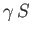, where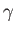only depends on the temperature and chemical composition of the two media on either side of the interface. It follows, from standard thermodynamics, thatis the work that must be performed on the system in order to create the interface via an isothermal and reversible process (Reif 1965). However, this work is exactly the same as that which we would calculate on the assumption that the interface is in a state of uniform constant tension per unit length. Thus,can be interpreted as both a free energy per unit area of the interface, and a surface tension. This tension is such that a force of magnitudeper unit length is exerted across any line drawn on the interface, in a direction normal to the line, and tangential to the interface. More information on surface tension can be found in Lamb 1928, and Batchelor 2000.

Surface tension originates from intermolecular cohesive forces. The average free energy of a molecule in a given isotropic medium possessing an interface with a second medium is independent of its position, provided that the molecule does not lie too close to the interface. However, the free energy is modified when the molecule's distance from the interface becomes less than the range of the cohesive forces (which is typically). Because this range is so small, the number of molecules in a macroscopic system whose free energies are affected by the presence of an interface is directly proportional to the interfacial area. Hence, the contribution of the interface to the total free energy of the system is also proportional to the interfacial area. If only one of the two media in question is a condensed phase then the parameteris invariably positive (i.e., such that a reduction in the surface area is energetically favorable). This follows because the molecules of a liquid or a solid are subject to an attractive force from neighboring molecules. However, molecules that are near to an interface with a gas lack neighbors on one side, and so experience an unbalanced cohesive force directed toward the interior of the liquid/solid. The existence of this force makes it energetically favorable for the interface to contract (i.e.,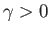). On the other hand, if the interface separates a liquid and a solid, or a liquid and another liquid, then the sign ofcannot be predicted by this argument. In fact, it is possible for both signs ofto occur at liquid/solid and liquid/liquid interfaces.

The surface tension of a water/air interface at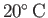is(Batchelor 2000). The surface tension at most oil/air interfaces is much lower--typically,(Batchelor 2000). On the other hand, interfaces between liquid metals and air generally have very large surface tensions. For instance, the surface tension of a mercury/air interface atis(Batchelor 2000).

For some pairs of liquids, such as water and alcohol, an interface cannot generally be observed because it is in compression (i.e.,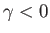). Such an interface tends to become as large as possible, leading to complete mixing of the two liquids. In other words, liquids for whichare immiscible, whereas those for whichare miscible.

Finally, the surface tension at a liquid/gas or a liquid/liquid interface can be affected by the presence of adsorbed impurities at the interface. For instance, the surface tension at a water/air interface is significantly deceased in the presence of adsorbed soap molecules. Impurities that tend to reduce surface tension at interfaces are termed surfactants.Next: Young-Laplace Equation Up: Surface Tension Previous: Surface Tension
Richard Fitzpatrick 2016-03-31